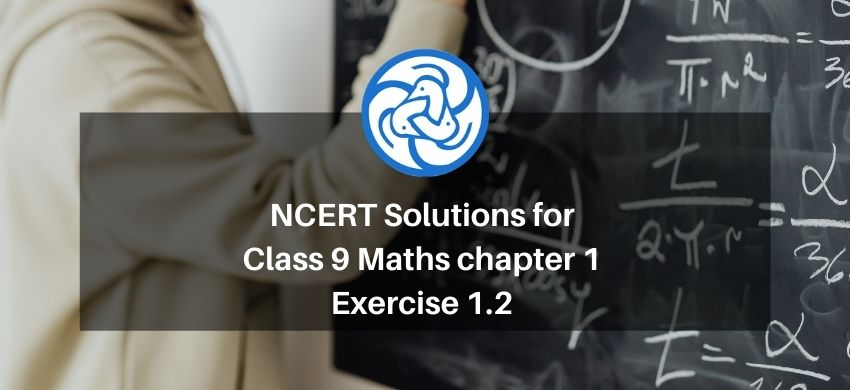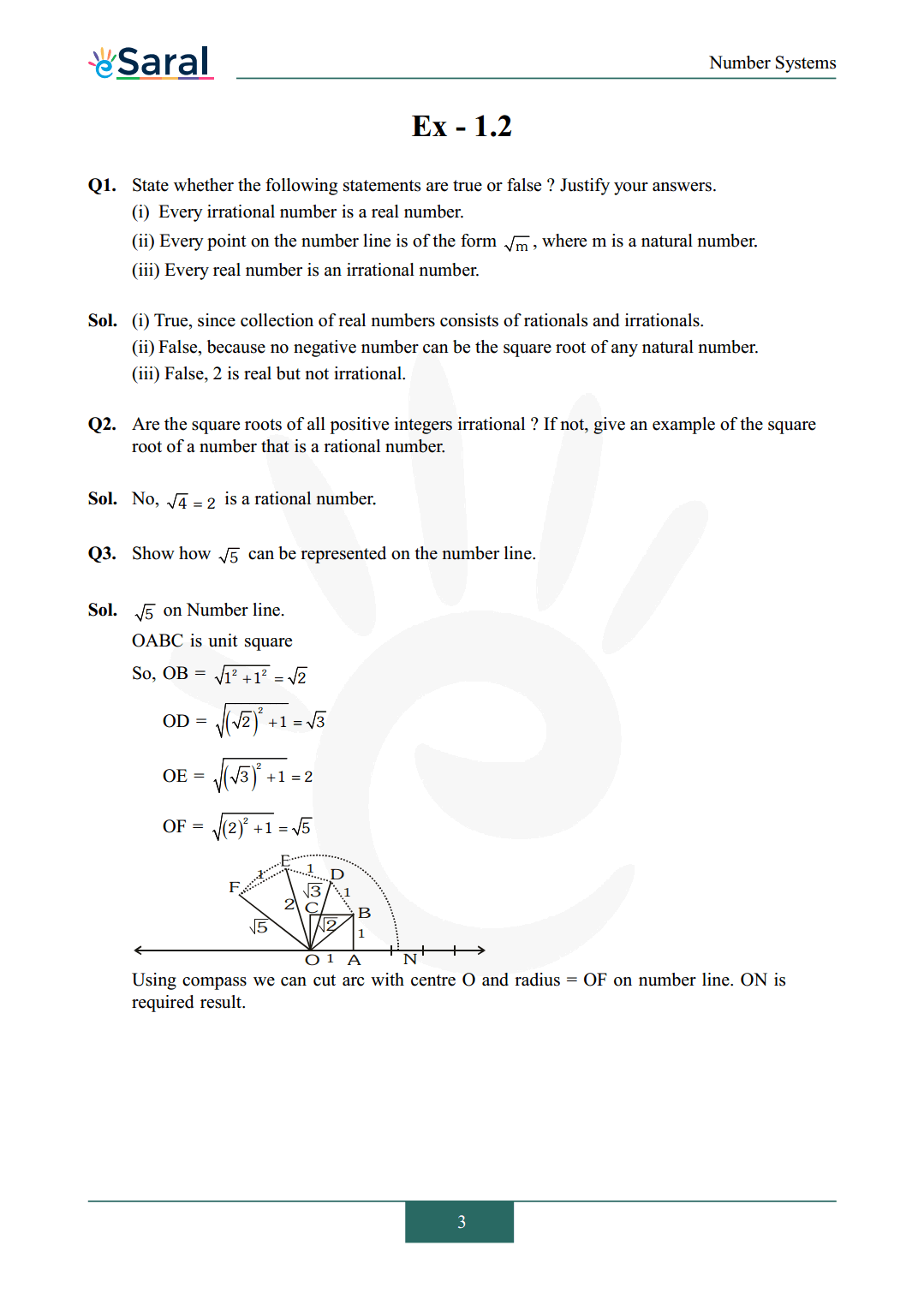Most Affordable JEE | NEET | 8,9,10 Preparation by Kota's Top IITian Doctor Faculties

# NCERT Solutions for Class 9 Maths chapter 1 Exercise 1.2 - Number System - Free PDF Download`
Hey, are you a class 9 Student and Looking for Ways to Download NCERT Solutions for Class 9 Maths chapter 1 Exercise 1.2? If Yes then you are at the right place.

Here we have listed Class 9 maths chapter 1 exercise 1.2 solutions  in PDF that is prepared by Kota’s top IITian’s Faculties by keeping Simplicity in mind.

If you want to score high in your class 9 Maths Exam then it is very important for you to have a good knowledge of all the important topics, so to learn and practice those topics you can use eSaral NCERT Solutions.

So, without wasting more time Let’s start.

### Download The PDF of NCERT Solutions for Class 9 Maths chapter 1 Exercise 1.2 "Number System"#### All Questions of Chapter 1 Exercise 1.2

Once you complete the chapter 1 then you can revise Ex. 1.2 by solving following questions

Q1. State whether the following statements are true or false ? Justify your answers.
(i) Every irrational number is a real number.
(ii) Every point on the number line is of the form $\sqrt{\mathrm{m}}$, where $\mathrm{m}$ is a natural number.
(iii) Every real number is an irrational number.

Q2. Are the square roots of all positive integers irrational ? If not, give an example of the square root of a number that is a rational number.

Q3. Show how $\sqrt{5}$ can be represented on the number line.

Class 9 Maths Short Revision Notes.

Class 9 NCERT Maths Book

Class 9 NCERT Maths Exemplar

Complete Solutions for Class 9 chapter 1

If you have any Confusion related to NCERT Solutions for Class 9 Maths chapter 1 Exercise 1.2 then feel free to ask in the comments section down below.

To watch Free Learning Videos on Class 9 by Kota’s top Faculties Install the eSaral App

Praveen
June 12, 2023, 5:21 a.m.
Exercise-1.2 , 1.3 , 1.4 , 1.5 question answer send me
Praveen
June 12, 2023, 5:35 a.m.
Nice
Priyanshu
June 6, 2022, 1:25 p.m.
Nice
Sathish Kumar
Nov. 23, 2021, 4:42 p.m.
Super
Priya
July 7, 2021, 8:37 a.m.